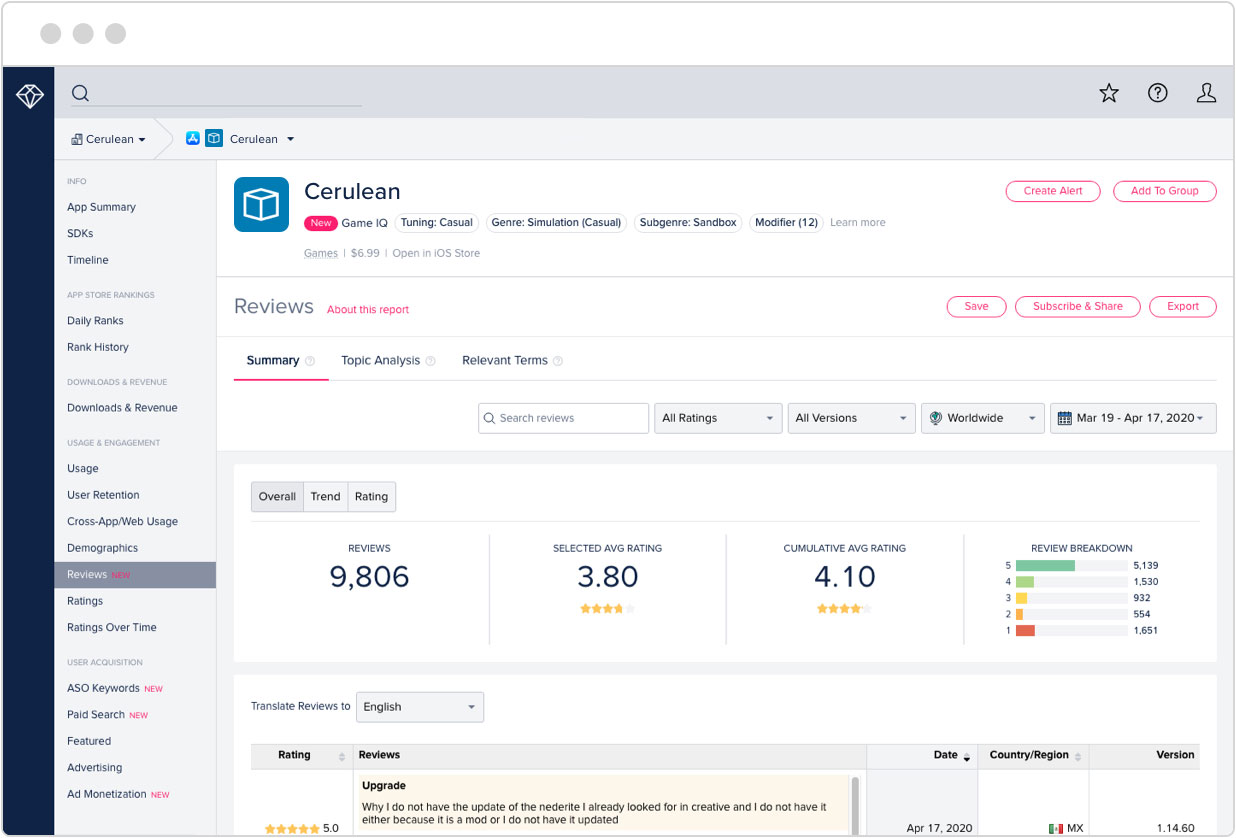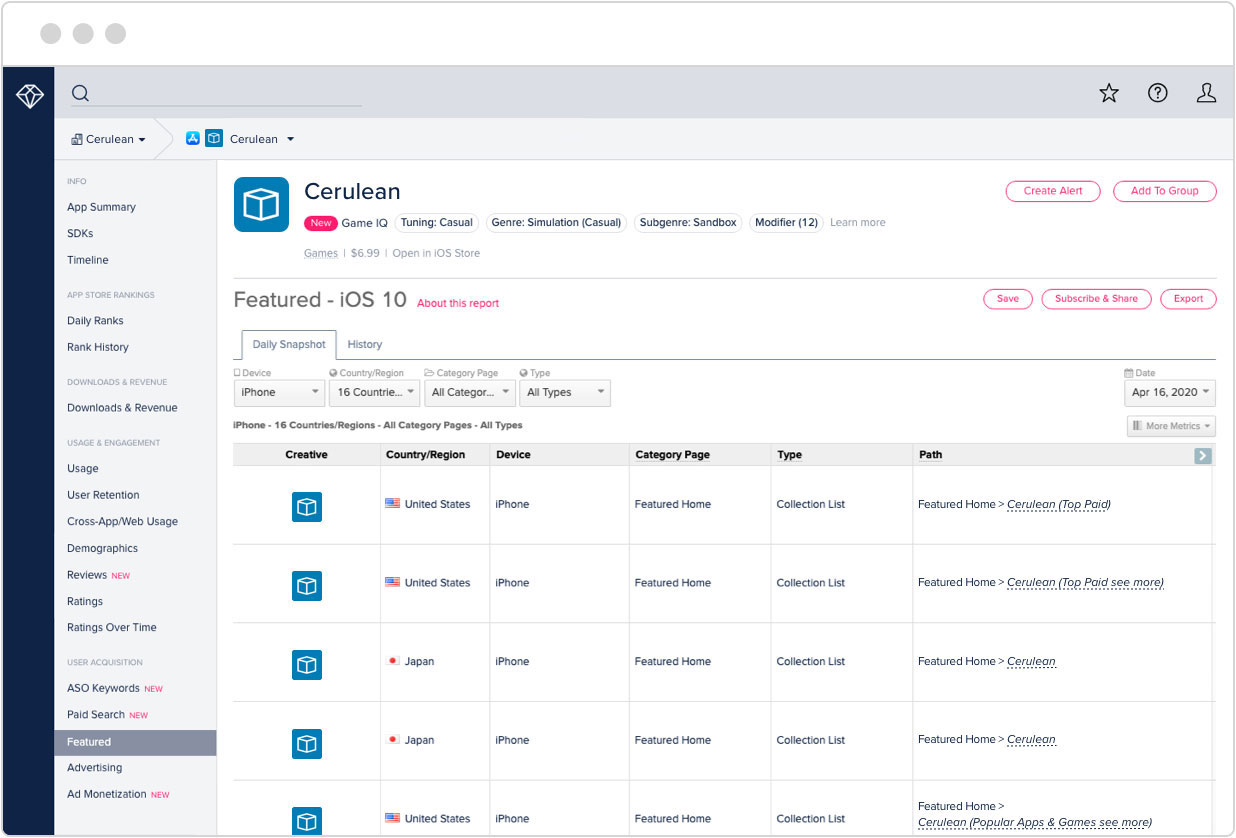Топ приложений

Искать любое приложение# Wolfram Multivariable Calculus Course Assistant

Издатель: Wolfram Group LLC
Цена: 4.99 дол. США
Оценка:

### История рейтингов приложения

Проверьте историю рейтингов загрузок Wolfram Multivariable Calculus Course Assistant в Россия.

История рейтингов показывает, насколько приложение Wolfram Multivariable Calculus Course Assistant популярно в магазине iOS, и позволяет узнать об изменениях популярности с течением времени. Вы можете отслеживать показатели эффективности Wolfram Multivariable Calculus Course Assistant по дням и по часам в разных странах, категориях и на разных устройствах.

### Узнайте больше после бесплатной регистрации!### Оптимизация для магазинов приложений

Отслеживайте топ ключевых слов для любого приложения и узнавайте, как его поисковая позиция меняется с течением времени благодаря их использованию.

### Отзывы и оценки приложения

Получайте ценные данные от ваших пользователей, изучая Отзывы и Оценки приложений. Делите данные по версиям, странам и датам, чтобы узнавать о багах, следить за интересными предложениями и улучшать свое приложение.### Реклама

Узнайте, где и когда приложения были рекомендованы в разных магазинах приложений с точностью до дня, страны, устройства и секции.

### Описание приложения

Taking multivariable calculus? Then you need the Wolfram Multivariable Calculus Course Assistant. This definitive app for multivariable calculus—from the world leader in math software—will help you work through your homework problems, ace your tests, and learn calculus concepts. Forget canned examples! The Wolfram Multivariable Calculus Course Assistant solves your specific multivariable problems on the fly, providing step-by-step guidance for limits, derivatives, integrals, and much more.

This app covers the following topics applicable to Multivariable Calculus, Advanced Calculus, and Vector Calculus:

- Evaluate any numeric expression, or substitute a value for a variable
- Plot 2D or 3D functions of your choice
- Determine the limit of a function as it approaches a specific value or values
- Differentiate any single or multivariable function
- Find the critical points and saddle points of a function
- Calculate the gradient of a function
- Identify the local extrema of a function
- Find the single, double, or triple integral of a function
- Determine the dot or cross product of two vectors
- Calculate the divergence or curl of a vector field

The Wolfram Multivariable Calculus Course Assistant is powered by the Wolfram|Alpha computational knowledge engine and is created by Wolfram Research, makers of Mathematica—the world's leading software system for mathematical research and education.

The Wolfram Calculus Course Assistant draws on the computational power of Wolfram|Alpha's supercomputers over a 3G, 4G, or Wi-Fi connection.

### Оптимизация для магазинов приложений

Какие слова люди используют, чтобы найти приложение? Правильные ключевые слова помогут повысить заметность приложения, а так же улучшить показатели загрузок и дохода. data.ai отслеживает миллионы ключевых слов, чтобы вы смогли увеличить количество загрузок своего приложения и понять, какие ключевые слова используют ваши конкуренты.

### Отзывы и оценки приложения

Узнайте, что пользователи думают о Wolfram Multivariable Calculus Course Assistant прочитав отзывы и просмотрев оценки этого приложения.